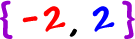OK, here's the trick: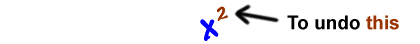We'll take the square root: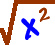Squares and square roots undo each other... Right?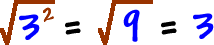Yep!

So,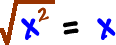Now, remember our equation rules -- whatever we do to one side, we have to do to the other.  So, we'll take the square root of both sides: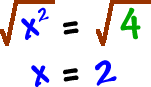Uh oh... We only got one answer.  Hmm... We got two answers when we factored.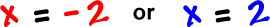Here's what you officially do to fix this problem: